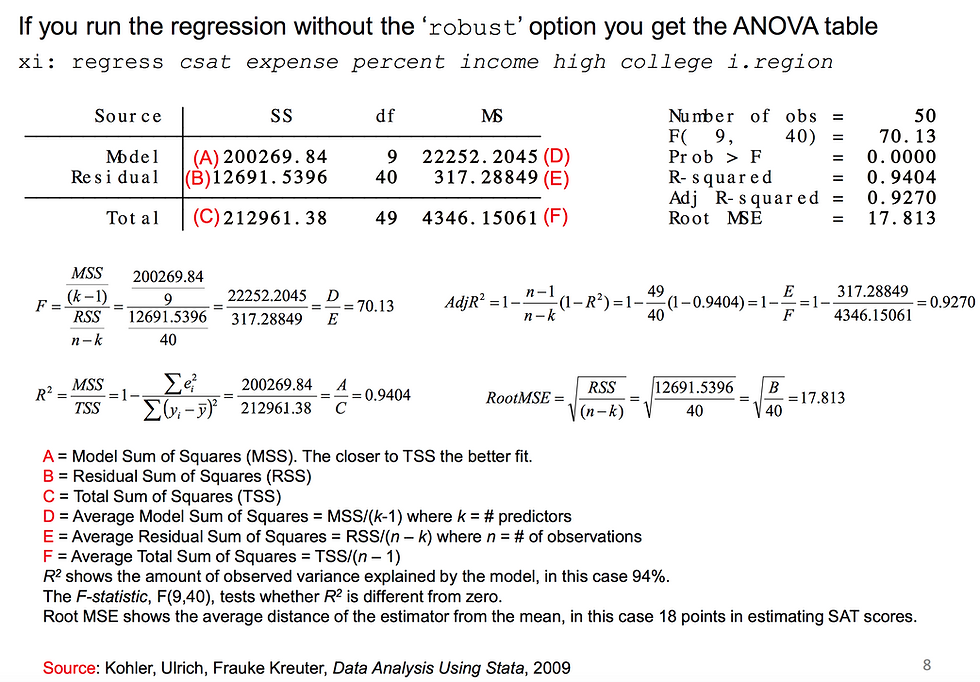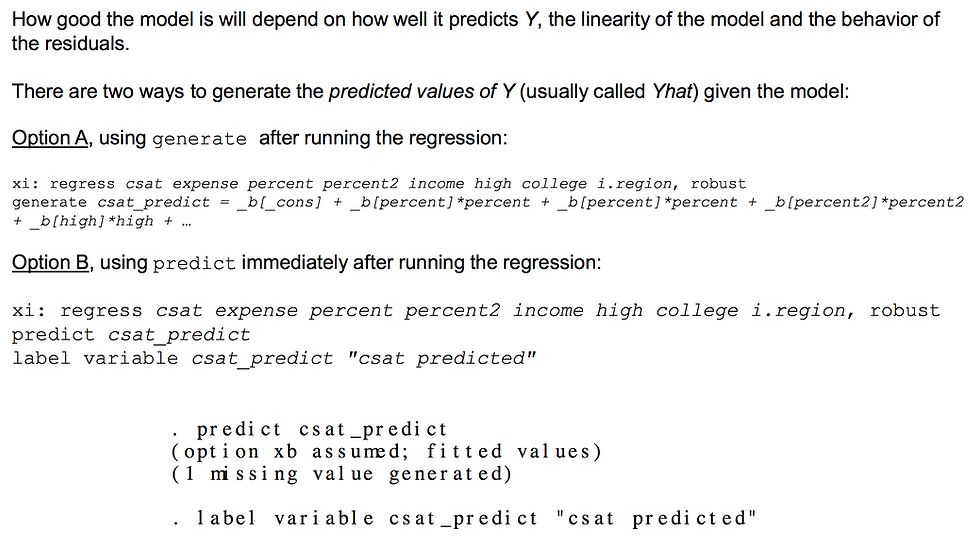top of page
Search

# REGRESSION WITH STATA - SIMPLE AND MULTIPLE REGRESSION

Multiple regression (an extension of simple linear regression) is used to predict the value of a dependent variable (also known as an outcome variable) based on the value of two or more independent variables (also known as predictor variables). For example, you could use multiple regression to determine if exam anxiety can be predicted based on coursework mark, revision time, lecture attendance and IQ score (i.e., the dependent variable would be "exam anxiety", and the four independent variables would be "coursework mark", "revision time", "lecture attendance" and "IQ score").

How to perform it in STATA:

In Stata use the command regress, type:

regress [dependent variable] [independent variable(s)]

In a multivariate setting we type:

regress y x1 x2 x3 ...